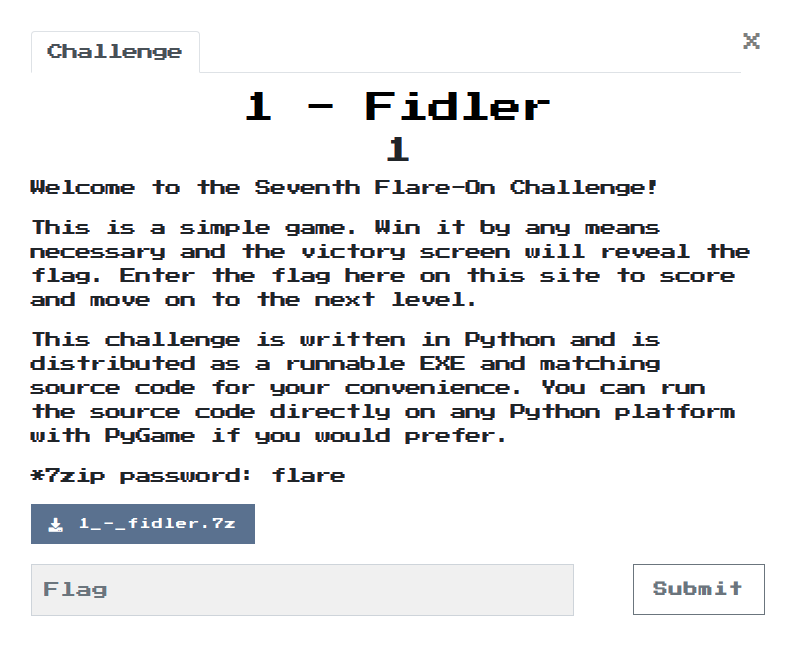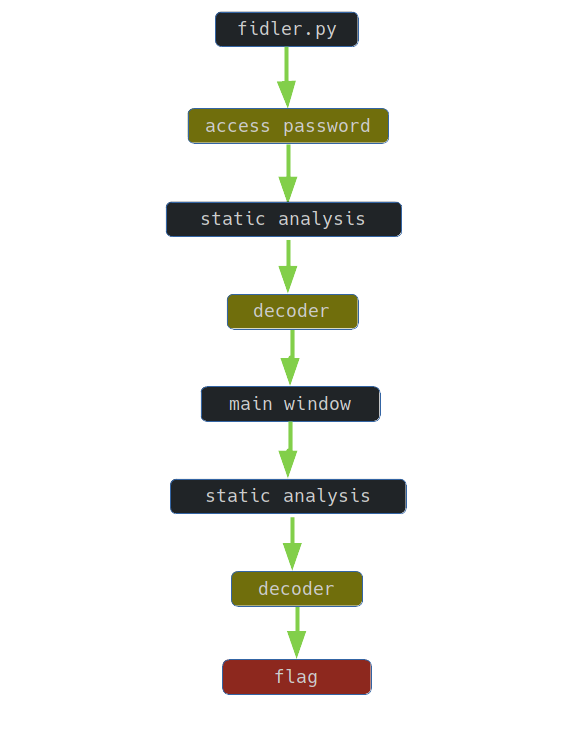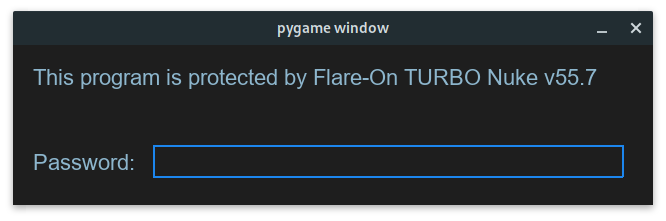# Writeup: FlareOn 2020: 001 - Fidler## 1. TLDR## 2. Input data

The challenge file is here. Password: flare.

The subject of the task is a program written in python. The available files are:

``````  controls.py
fidler.py
fonts/arial.ttf
fonts/courbd.ttf
img/btndown.png
img/btnup.png
img/clock.png
img/coin.png
img/fbi.png
img/kittyelaine.png
Message.txt
fidler.exe
``````

Due to the fact that the program logic is implemented in the file `fidler.py`, it is enough to analyze this file.The program is written in python, its code is immediately available.

The `password_check()` function is responsible for verifying the password:

``````def password_check(input):
altered_key = 'hiptu'
key = ''.join([chr(ord(x) - 1) for x in altered_key])
return input == key
``````

In order to obtain the password, it is enough to execute the script:

``````altered_key = 'hiptu'
key = ''.join([chr(ord(x) - 1) for x in altered_key])
print(key)
``````

Thus, on the standard output, you can get the password written out, which allows you to access the program:

``````ghost
``````

## 4. Code analysis

After entering the access password, the appropriate application window appears:The following functions are responsible for reading and presenting the flag:

• decode_flag:
``````def decode_flag(frob):
last_value = frob
encoded_flag = [1135, 1038, 1126, 1028, 1117, 1071, 1094, 1077, 1121, 1087, 1110, 1092, 1072, 1095, 1090, 1027,
1127, 1040, 1137, 1030, 1127, 1099, 1062, 1101, 1123, 1027, 1136, 1054]
decoded_flag = []

for i in range(len(encoded_flag)):
c = encoded_flag[i]
val = (c - ((i%2)*1 + (i%3)*2)) ^ last_value
decoded_flag.append(val)
last_value = c

return ''.join([chr(x) for x in decoded_flag])
``````
• victory_screen:
``````def victory_screen(token):
...
flag_content_label.change_text(decode_flag(token))
...
``````
• game_screen:
``````def game_screen():
...
while not done:
target_amount = (2**36) + (2**35)
if current_coins > (target_amount - 2**20):
while current_coins >= (target_amount + 2**20):
current_coins -= 2**20
victory_screen(int(current_coins / 10**8))
return
...
``````

Therefore, in order to read the flag, the code should be executed:

``````#! /usr/bin/python3

def decode_flag(frob):
last_value = frob
encoded_flag = [1135, 1038, 1126, 1028, 1117, 1071, 1094, 1077, 1121, 1087, 1110, 1092, 1072, 1095, 1090, 1027,
1127, 1040, 1137, 1030, 1127, 1099, 1062, 1101, 1123, 1027, 1136, 1054]
decoded_flag = []

for i in range(len(encoded_flag)):
c = encoded_flag[i]
val = (c - ((i%2)*1 + (i%3)*2)) ^ last_value
decoded_flag.append(val)
last_value = c

return ''.join([chr(x) for x in decoded_flag])

def victory_screen(token):
print(decode_flag(token))

def main():
target_amount = (2**36) + (2**35)
current_coins = (target_amount - 2**20) + 1
while current_coins >= (target_amount + 2**20):
current_coins -= 2**20
victory_screen(int(current_coins / 10**8))

if __name__ == "__main__":
main()
``````

The flag is printed on the standard output:

``````idle_with_kitty@flare-on.com
``````